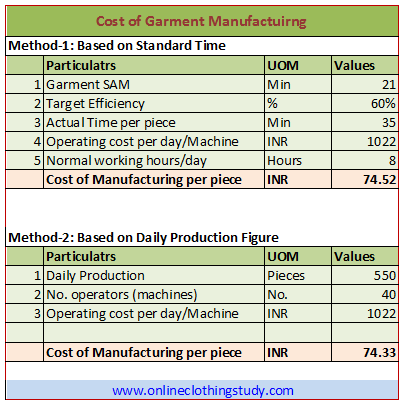# How to Calculate Cost of Manufacturing Apparel Products

Estimating correct manufacturing cost is essential for a successful business. You might be preparing a garment cost sheet and need to find manufacturing cost per garment. But don't know exactly how to do it and don't have full confidence calculating the same. Don't worry.

Read this post and learn the basic method of determining the Cost of Manufacturing (CM) of apparel products.

### What is the cost of manufacturing

Cost of manufacturing can be defined as the cost incurred by the factory to run the factory making garments. Factory running cost includes direct labour cost and manufacturing overhead. Here manufacturing overheads are indirect workers wages, staff salary, power and fuel cost, repair and maintenance cost, factory rent, administration cost etc. This cost is also known as an operating cost. Cost of Manufacturing is determined for per unit garment.

There are two ways to find the Cost of Manufacturing (CM) for a particular style/order.
1.  Based on Standard Time (SAM) of the product
2.  Based on Daily Production Average

### 1. CM Calculation Based on Standard Time (SAM) of Product Making

To get better accuracy in cost estimation one should prefer this procedure. Many small-size companies do not have such resources to measure product SAM and data for the following parameters. Following parameters are essential for cost calculation in this method.
1. Product SAM: Standard time of the garment. The standard time of a garment is measured by using Time Study and using synthetic data
2. Target Efficiency:  Target efficiency percentage is at what % you are expecting running a specific product and order quantity.
3. Operating Cost per day/machine - Operating cost is factory running cost. Operating costs are all cost incurred to run the business other than material cost. Calculate monthly operating cost and then calculate the daily operating cost. Calculate per machine operating cost.
Formula:
Cost of Manufacturing = (Operating cost per day per machine* SAM)/(Target Efficiency% * Working hours * 60)
In the following table, an example is shown for calculating manufacturing cost using SAM and Daily Production figure.Table-1: Calculation of Cost of Manufacturing
In the above example, garment SAM is 21 minute, target efficiency 60%. So, the actual time would be 35 minutes to make a garment. The factory works 8 hours a day and operating cost per day per machine is Rs. 1022.

Cost of manufacturing is Rs. 74.52

### 2. CM Calculation Based on Daily Production Figure

This method is widely used by garment manufacturing factories. Cost of Manufacturing calculation is done based on historical production data. This is an easier method compared to the above one.

Information needed to find Cost of Manufacturing
1. Daily production: Find the average daily production of a particular style (garment) based on earlier (historical) production figures. Calculate the daily average production of the factory.
2. Manpower involved in production: How many sewing machines or sewing operators are utilized to produce the above quantity.
3. Operating cost per day/machine: As explained above.
Formula,
Cost of Manufacturing = (Operating cost per Day / Total garments to be produced per day)
See the example method -2 in the above table. In the example, the daily production is 550 pieces. 40 operators worked to produce these pieces. Operating cost per machine is Rs. 1022 per day.

So the cost of manufacturing is Rs. 74.33

P.S. Data used in the above examples are hypothetical.

Also read: Actual Garment Production Cost -the way factory calculates it